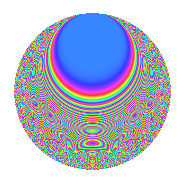# Properties

 Label 731.2.eLevel 731 Weight 2 Character orbit e Rep. character $$\chi_{731}(307,\cdot)$$ Character field $$\Q(\zeta_{3})$$ Dimension 116 Newforms 2 Sturm bound 132 Trace bound 2

# Related objects

## Defining parameters

 Level: $$N$$ = $$731 = 17 \cdot 43$$ Weight: $$k$$ = $$2$$ Character orbit: $$[\chi]$$ = 731.e (of order $$3$$ and degree $$2$$) Character conductor: $$\operatorname{cond}(\chi)$$ = $$43$$ Character field: $$\Q(\zeta_{3})$$ Newforms: $$2$$ Sturm bound: $$132$$ Trace bound: $$2$$ Distinguishing $$T_p$$: $$2$$

## Dimensions

The following table gives the dimensions of various subspaces of $$M_{2}(731, [\chi])$$.

Total New Old
Modular forms 136 116 20
Cusp forms 128 116 12
Eisenstein series 8 0 8

## Trace form

 $$116q - 4q^{2} - 2q^{3} + 108q^{4} - 4q^{5} + 4q^{6} - 6q^{7} - 52q^{9} + O(q^{10})$$ $$116q - 4q^{2} - 2q^{3} + 108q^{4} - 4q^{5} + 4q^{6} - 6q^{7} - 52q^{9} + 8q^{10} + 16q^{11} + 4q^{12} + 4q^{13} - 6q^{14} + 2q^{15} + 60q^{16} + 24q^{18} + 4q^{19} - 40q^{20} - 52q^{21} - 32q^{22} - 16q^{24} - 60q^{25} - 8q^{26} + 16q^{27} - 10q^{28} + 12q^{29} + 6q^{30} + 6q^{31} - 24q^{32} - 6q^{33} - 4q^{34} + 12q^{35} - 46q^{36} - 14q^{37} + 2q^{38} + 76q^{39} + 20q^{40} - 8q^{41} + 38q^{43} - 44q^{45} - 74q^{46} - 16q^{47} + 22q^{48} - 64q^{49} - 30q^{50} + 16q^{51} + 50q^{52} - 20q^{53} + 24q^{54} + 16q^{55} - 58q^{56} - 14q^{57} - 14q^{58} - 24q^{59} + 24q^{60} - 36q^{61} + 2q^{62} - 36q^{63} + 56q^{64} + 52q^{65} - 60q^{66} - 6q^{67} - 34q^{69} + 12q^{70} + 10q^{71} + 38q^{72} + 42q^{74} + 16q^{75} + 14q^{77} - 96q^{78} - 14q^{79} - 104q^{80} - 2q^{81} + 8q^{82} + 6q^{83} + 64q^{84} + 4q^{85} - 32q^{86} + 16q^{87} - 56q^{88} + 28q^{89} + 20q^{90} - 38q^{91} - 24q^{92} + 184q^{94} + 26q^{95} - 40q^{96} + 56q^{97} + 28q^{98} - 34q^{99} + O(q^{100})$$

## Decomposition of $$S_{2}^{\mathrm{new}}(731, [\chi])$$ into irreducible Hecke orbits

Label Dim. $$A$$ Field CM Traces $q$-expansion
$$a_2$$ $$a_3$$ $$a_5$$ $$a_7$$
731.2.e.a $$58$$ $$5.837$$ None $$-6$$ $$3$$ $$-1$$ $$7$$
731.2.e.b $$58$$ $$5.837$$ None $$2$$ $$-5$$ $$-3$$ $$-13$$

## Decomposition of $$S_{2}^{\mathrm{old}}(731, [\chi])$$ into lower level spaces

$$S_{2}^{\mathrm{old}}(731, [\chi]) \cong$$ $$S_{2}^{\mathrm{new}}(43, [\chi])$$$$^{\oplus 2}$$